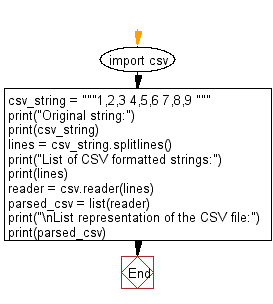﻿ Python: Parse a given CSV string and get the list of lists of string values - w3resource# Python: Parse a given CSV string and get the list of lists of string values

## Python module: Exercise-3 with Solution

Write a Python program to parse a given CSV string and get the list of lists of string values. Use csv.reader

We will get a list of lists of string values after parsing a CSV formatted string where each list of strings represents a line. For example "x, y, z" will become [["x", "y", "z"]].

Sample Solution:

Python Code:

``````import csv
csv_string = """1,2,3
4,5,6
7,8,9
"""
print("Original string:")
print(csv_string)
lines = csv_string.splitlines()
print("List of CSV formatted strings:")
print(lines)
print("\nList representation of the CSV file:")
print(parsed_csv)
```
```

Sample Output:

```Original string:
1,2,3
4,5,6
7,8,9

List of CSV formatted strings:
['1,2,3', '4,5,6', '7,8,9']

List representation of the CSV file:
[['1', '2', '3'], ['4', '5', '6'], ['7', '8', '9']]
```

Flowchart:Python Code Editor:

Have another way to solve this solution? Contribute your code (and comments) through Disqus.

What is the difficulty level of this exercise?

Test your Python skills with w3resource's quiz

﻿

## Python: Tips of the Day

For-else construct useful when searched for something and find it:

```# For example assume that I need to search through a list and process each item until a flag item is found and
# then stop processing. If the flag item is missing then an exception needs to be raised.

for i in mylist:
if i == theflag:
break
process(i)
else:
raise ValueError("List argument missing terminal flag.")
```